# PSAT Math : How to use FOIL with Exponents

## Example Questions

### Example Question #1161 : Algebra

If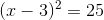, which of the following could be the value of?Explanation:Take the square root of both sides.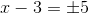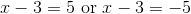Add 3 to both sides of each equation.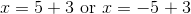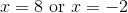### Example Question #1 : How To Use Foil With Exponents

Simplify: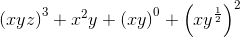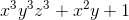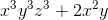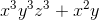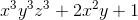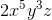Explanation:= x3y3z3 + x2y + x0y0 + x2y

x3y3z3 + x2y + 1 + x2y

x3y3z3 + 2x2y + 1

### Example Question #4 : Exponents And The Distributive Property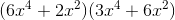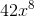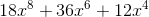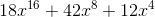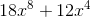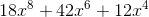Explanation:

Use the FOIL method to find the product.  Remember to add the exponents when multiplying.First: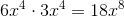Outside: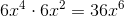Inside: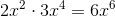Last: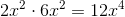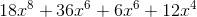### Example Question #9 : How To Use Foil With Exponents

Square the binomial.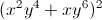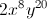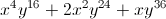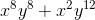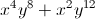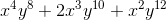Explanation: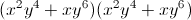We will need to FOIL.

First: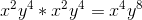Inside: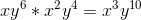Outside: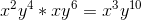Last: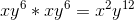Sum all of the terms and simplify.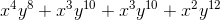### Example Question #121 : Exponents

Which of the following is equivalent to 4c(3d)– 8c3d + 2(cd)4?

cd(54c * d– 4c+ c* d2)

2cd(54d2 – 4c+ c* d3)

2(54d– 4c+ 2c* d3)